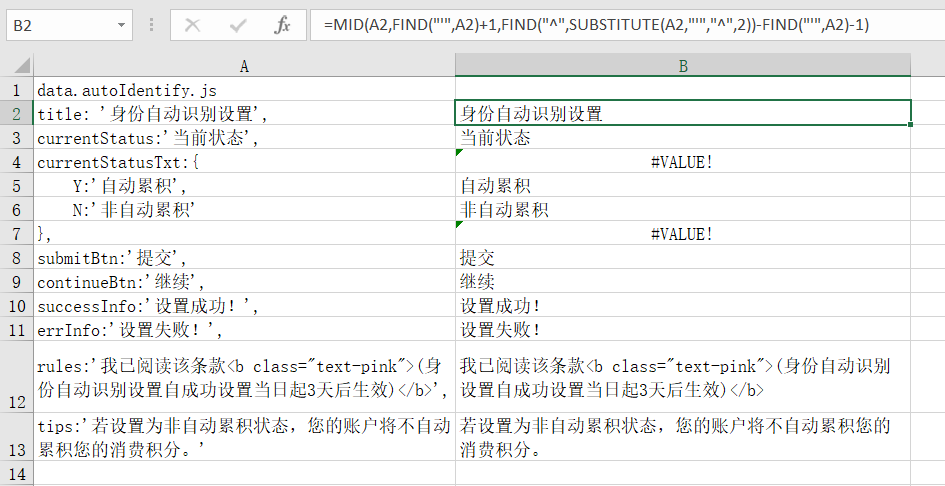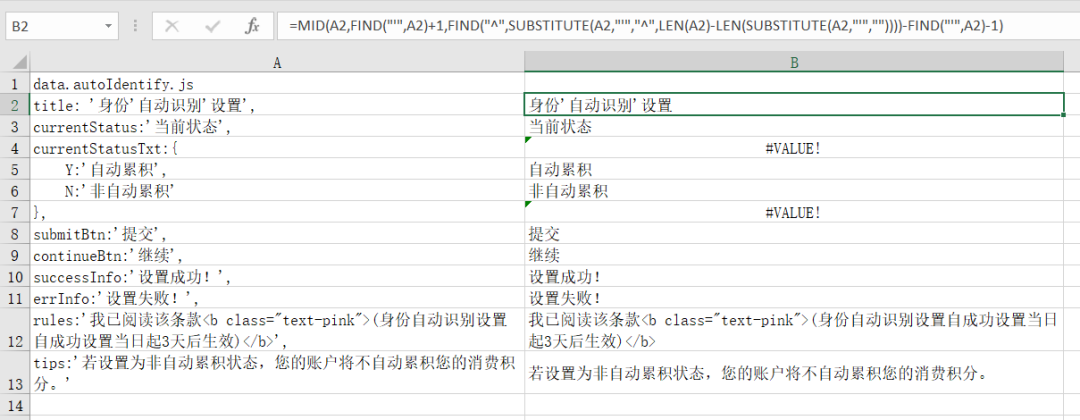# 【Excel】用公式提取Excel单元格中的汉字

### 一、用公式提取Excel单元格中的汉字=LEFT(A2,LENB(A2)-LEN(A2))

=RIGHT(A6,LENB(A6)-LEN(A6))

=MID(A10,MATCH(2,LENB(MID(A10,ROW(INDIRECT("1:"&LEN(A10))),1)),),LENB(A10)-LEN(A10))

1. 按Alt+F11，打开VBA编辑器，单击菜单“插入→模块”，在代码窗口中输入粘贴下面的代码：

Function 提取汉字(sString As String) As String

Dim regEx As Object

Set regEx = CreateObject("VBScript.RegExp")

With regEx

'搜索整个字符串

.Global = True

'匹配非汉字

.Pattern = "[^\u4e00-\u9fa5]"

'将字符串中的非汉字替换为空

End With

Set regEx = Nothing

End Function

2. 返回Excel工作表界面，在B14单元格中输入公式：

=提取汉字(A14)

### 二、用公式提取引号（某2个相同字符）之间的内容=MID(A2,FIND("'",A2)+1,FIND("^",SUBSTITUTE(A2,"'","^",LEN(A2)-LEN(SUBSTITUTE(A2,"'",""))))-FIND("'",A2)-1)

=FIND("这个不重复就行",SUBSTITUTE(A1,"-"," 这个不重复就行",LEN(A1)-LEN(SUBSTITUTE(A1,"-",""))))

=find("这个不重复就行",substitute(A1,"\","这个不重复就行",4))

### 三、用FIND函数查找字符串中的双引号

=FIND("""",E11)

03-223253
10-251213
05-08
10-18
11-091万+
11-163468
11-091392
09-18
12-211988
01-271022
08-27573
12-16320
12-04105
07-292万+
02-081257
03-131万+

### “相关推荐”对你有帮助么？

•非常没帮助
•没帮助
•一般
•有帮助
•非常有帮助

©️2022 CSDN 皮肤主题：大白 设计师：CSDN官方博客被折叠的  条评论 为什么被折叠?到【灌水乐园】发言点击重新获取扫码支付1.余额是钱包充值的虚拟货币，按照1:1的比例进行支付金额的抵扣。
2.余额无法直接购买下载，可以购买VIP、C币套餐、付费专栏及课程。余额充值